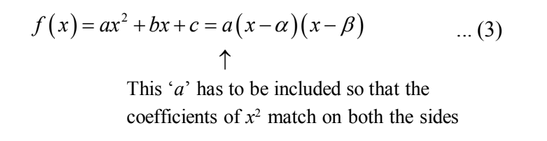# Examples On Basic Quadratic Problems Set-1

Example- 1

Find the roots of

(a) $$f\left( x \right) = {x^2} + 3x + 2$$       (b) $$f\left( x \right) = {a^2}{x^2} + 2abx + {b^2}$$       (c) $$f\left( x \right) = {x^2} + x + 1$$

Solution (a)  $$f\left( x \right)$$ can be factorized:

${x^2} + 3x + 2 = \left( {x + 1} \right)\left( {x + 2} \right)$

This is 0 if $$x = - 1,\, - 2$$

Alternatively, we could use the quadratic formula for the roots:

\begin{align}&{x_1},{x_2}\,\, = \frac{{ - 3 \pm \sqrt {9 - 4 \times 1 \times 2} }}{2}\\\,\,\,\,\,\,\,\,\,\,\,\, &\qquad\quad= \frac{{ - 3 \pm 1}}{2}\\\,\,\,\,\,\,\,\,\,\,\,\, &\qquad\quad= - 1, - 2\end{align}

(b) $$f\left( x \right)$$ here is a perfect square:

$f\left( x \right) = {a^2}{x^2} + 2abx + {b^2} = {\left( {ax + b} \right)^2}$

This is evidently 0 when

\begin{align}&\,\,\,\,\,\,\,\,\,\,ax + b = 0\\ &\Rightarrow x = \frac{{ - b}}{a}\end{align}

We see that $$f\left( x \right)$$ has equal roots. The parabola for $$f\left( x \right)$$ touches the x-axis.

(c) $$f\left( x \right)$$ can be rearranged as

${x^2} + x + 1 = {\left( {x + \frac{1}{2}} \right)^2} + \frac{3}{4}$

(by following the general outline on page - 2)

We see that $$f\left( x \right) > 0$$  for all values of x.

$$\Rightarrow f\left( x \right)$$ has no real roots

The complex roots can be obtained from the quadratic formula:

\begin{align}&{x_1},{x_2} = \frac{{ - 1 \pm \sqrt {1 - 4} }}{2}\\\,\,\,\,\,\,\,\,\,\,\,\, &\qquad\;\;= \frac{{ - 1 \pm \sqrt { - 3} }}{2}\end{align}

Example- 2

Solve the following inequalities for x:

(a) $${x^2} + 3x + 2 > 0$$           (b) $${x^2} + 3x + 5 < 0$$                (c) $${x^2} + 2x + 1 > 0$$

Solution:   We saw how to solve such inequalities in the previous section. We urge you to recall that discussion and apply it on these examples by actually drawing the corresponding graphs.

(a)   $${x^2} + 3x + 2 > 0$$

$$\begin{array}{l}\\ \Rightarrow \left( {x + 1} \right)\left( {x + 2} \right) > 0\\ \Rightarrow x < - 2\,\,{\rm{or}}\,\,x > - 1\end{array}$$

(b)       $$\Rightarrow {\left( {x + \frac{3}{2}} \right)^2} + \frac{{11}}{4} < 0$$

$$\Rightarrow$$ This is never possible

$$\Rightarrow$$ No value of x satisfies the given inequality

Alternatively, we evaluate the expression’s discriminant:

$D = {b^2} - 4ac = 9 - 20 = - 11$

Since $$D < 0,$$ the given expression has no real roots so its parabola lies entirely above the x-axis $$f\left( x \right)$$ (is always positive) or in other words, for no value of x does the graph of lie below $$f\left( x \right)$$ the x-axis. Hence, no value of x satisfies this inequality

(c)         $${x^2} + 2x + 1 > 0$$

$$\begin{array}{l} \Rightarrow {\left( {x + 1} \right)^2} > 0\end{array}$$

We see that the inequality above is always true except the point(s) where the left hand side could become 0.

$$\Rightarrow \qquad x \ne - 1$$

$$\Rightarrow \qquad x \in \mathbb{R}\backslash \left\{ { - 1} \right\}$$

Example- 3

If $$\alpha ,\beta$$ are the roots of $$\left( {x - a} \right)\left( {x - b} \right) = c,$$  find the roots of $$\left( {x - \alpha } \right)\left( {x - \beta } \right) + c = 0$$

Solution:   Before solving this equation, we note that if $$f\left( x \right) = a{x^2} + bx + c = 0$$ has two roots $$\alpha$$  and $$\beta$$ then

$$f\left( \alpha \right) = f\left( \beta \right) = 0$$ so that $$\left( {x - \alpha } \right)\,\,{\rm{and}}\,\,\left( {x - \beta } \right)$$  are factors of $$f\left( x \right)$$(by the factor theorem)

Hence $$f\left( x \right)$$ , can be written asNow, in the current question $$\alpha ,\,\,\beta$$ , since are the roots of $$\left( {x - a} \right)\left( {x - b} \right) = c,$$ we can write

$\left( {x - a} \right)\left( {x - b} \right) - c = \left( {x - \alpha } \right)\left( {x - \beta } \right)$

This immediately gives

$\left( {x - \alpha } \right)\left( {x - \beta } \right) + c = \left( {x - a} \right)\left( {x - b} \right)$

The left hand side is the equation we wanted to solve. Since it is equal to $$\left( {x - a} \right)\left( {x - b} \right),$$ the required roots are a, b!

Example- 4

Let $$M\left( x \right) = p{x^2} + qx + r\,\,{\rm{and}}\,\,N\left( x \right) = - p{x^2} + sx + r.$$ Given that $$pr \ne 0,$$ show that $$M\left( x \right)N\left( x \right) = 0$$ will have at least two real roots.

Solution:   Note that since $$M\left( x \right)N\left( x \right)$$  will be a 4th degree polynomial, it will have four roots. These roots could be all real, all non-real or two real and two imaginary. (Why?)

Here we need to show that $$M\left( x \right)N\left( x \right)$$ will have at least two real roots, that is, all the roots will not be imaginary.

We need not consider the case when all the four roots are real.

Let us suppose that $$M\left( x \right)$$ has imaginary roots.

This means that

$${q^2} - 4pr < 0$$

or

$$4pr > {q^2}$$

The discriminant for $$N\left( x \right)$$ is

$$D = {s^2} + 4pr > {s^2} + {q^2}$$ (using 4pr > q2)

Hence, the D for N(x) is positive or N(x) will have two real roots.

Similarly, if N(x) has imaginary roots, M(x) will have real roots.

Thus, in all cases, $$M\left( x \right)N\left( x \right)$$ will have at least two real roots.

Learn from the best math teachers and top your exams

• Live one on one classroom and doubt clearing
• Practice worksheets in and after class for conceptual clarity
• Personalized curriculum to keep up with school